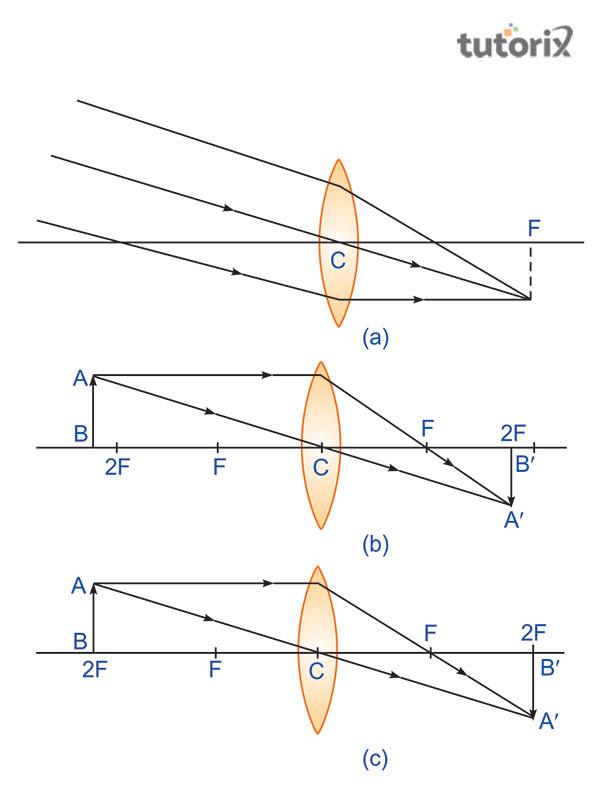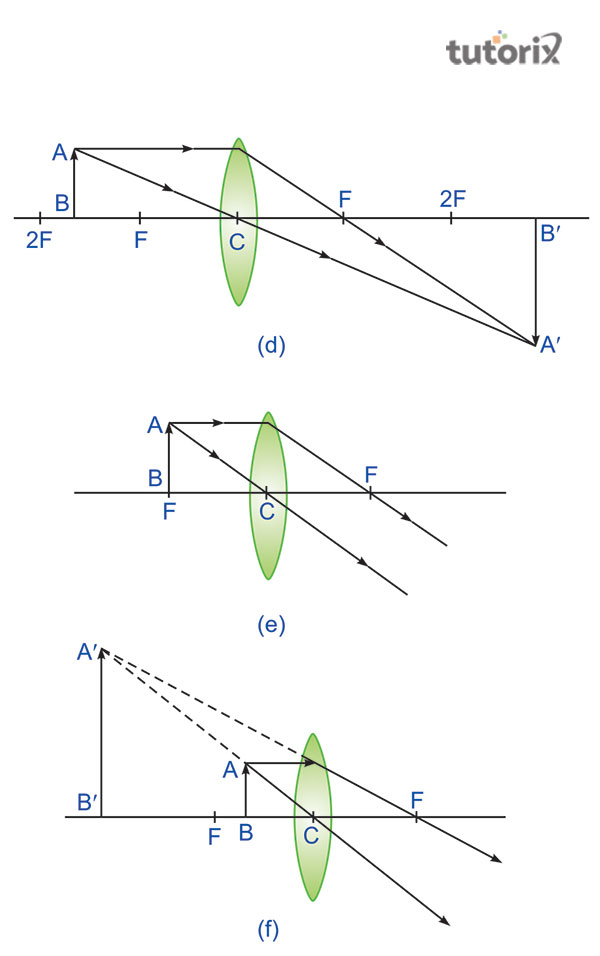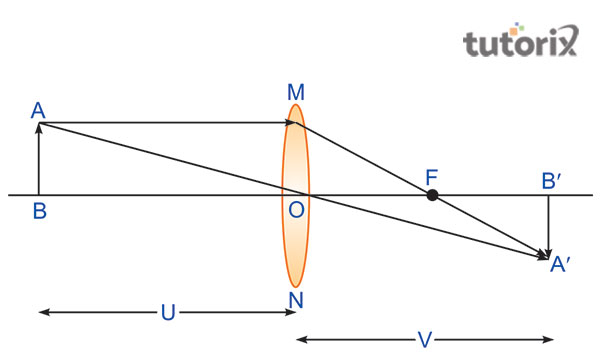# To find image distance for varying object distances of a convex lens with ray diagrams

In the study of optics, a lens is defined as a piece of glass or transparent substance which is used to form an image. Moreover, it is bounded by two surfaces. It forms images of any object just by focusing on the light, that comes from that particular object. It has several properties, such as the principal axis, principal focus, center of curvature of the lens, aperture and optical center. Between the two types of lens, here the convex lens is going to use in the experiment and it forms images that can be either a real or virtual image. Through this experiment, the image distance for varying object distances of a convex lens with ray diagrams can be found.

## Aim

This experiment aims to find the image distance in case of convex lens for any varying object and with the use of ray diagram can be shown how the nature of the image is formed.

## Theory

### A convex lens

The structure of a convex lens is thick at the center and thin from the edges. It can be defined as a lens with a surface that is bent outwards (Mao et al. 2022). A convex lens is also known as a biconvex lens or converging lens. This type of lens converges the light beam incident on it. Mainly 3 types of convex lens can be found, double, Plano and concavo.

### The formula of the lens

The relationship between the object distance [denoted as ‘u’], the distance of image [denoted as ‘v’] and the focal length [denoted as ‘f’] is referred to as the formula of the lens or as the lens formula (openstax, 2022). It can be shown as,

[1/f] = [(1/v)- (1/u)]

In this equation, the f= focal length, v= distance of the image that is from the lens's optical center and u= the distance between an object and lens’s optical center.

## Required material

In the process of conducting the experiment, few materials are mandatory (teachoo, 2022). A convex lens is required that has a focal length of 12 to 20 cm, for taking measurement a measurement scale, optical bench, a needle and most importantly a candle.

## ProcessFigure 1: Position of the objects

1. If at infinity u=∞, v will be equal to the f, which denotes the focal length, i. e v= f. As shown in the image (a), when light rays travel paralleled to each other, they form images on the other side. This image is formed on the other side of the lens and appears in a real, small and inverted way.

2. If any object can be placed beyond 2F, as a result, the image is going to be formed between F and 2F. As shown in image (b) the image will be smaller and inverted and formed on the opposite side of the lens.

3. If the object is placed at point 2F, u will be double the focal length and the same thing will happen to the v. As the image (c) shows, the formed image will be on the other side of the lens, of the same size as the object but inverted.Figure 2: position and image of the objects

4.If the object is placed just in between the F and 2F the image, that will beformed is going to be real, inverted and larger than the size of the object. It can be shown in the image (d).

5. If u is equal to the f, and the object is placed at the point F, the image will be formed on the other side of the lens and will be as real, inverted and extremely larger in size . Image (e) shows how this works.

6. A object can be placed in between the points C and F. In that case, the formed image will be erect, virtual larger in size and for a change on the same side of the lens (studyrankers, 2022). The most negative thing about this image is that it cannot be presented on-screen. It can be seen in image (f).

## Ray DiagramFigure 3: Ray Diagram

## Observation

No. Position of the optical center of the lens (cm) Position of candle (cm) The exact position of the screen (cm) Object distance (cm) Image Distance (cm) Focal length (cm)

Table 1: Observation through lens

## Calculation

That memes the focal length will be 10 cm. In short, the focal length of the given lens (figure 3) is 10 cm.

## Conclusion

This experiment demands some precautions, such as the focal length has to be in between 15 to 20cm, it must have a small aperture, and most importantly the screen should not be shaky. The image that is formed by a convex lens will always be real and inverted and will be seen on the other side. Only one time this will not happen if the object is placed in between the point F and C. In this position, the image that is going to be formed will be a virtual and enlarged version of the object, and most importantly it will be on the same side of the lens.

## FAQ

Q1. What is a convex lens?

A lens that has a surface which is bent outwards is called a convex lens. It is also known as the converge lens or biconvex lens.

Q2. What is the formula of the lens?

The lens formula is [1/f] = [(1/v)- (1/u)], where, f= focal length, v= object distance, u=image distance.

Q3. What is a focal length?

The focal length of a lens is the distance between principal focus and the optical center of the lens.